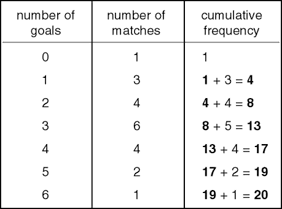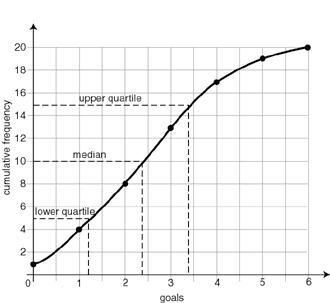Definition: quartile from BUSINESS: The Ultimate Resource

any of the values in a frequency or probability distribution that divide it into four equal parts

Summary Article: quartile
From The Hutchinson Unabridged Encyclopedia with Atlas and Weather Guide

In statistics, any one of the three values that divide data into four equal parts. They comprise the lower quartile, below which lies the lowest 25% of the data; the median, which is the middle 50%, half way through the data; and the upper quartile, above which lies the top 25%. The difference of value between the upper and lower quartiles is known as the interquartile range, which is a useful measure of the dispersion of a statistical distribution because it is not affected by freak extreme values (see range). These values are usually found using a cumulative frequency diagram.

For example, a football team scored the following goals in one season:This information can be displayed in a cumulative frequency curve:From the curve, the median is 2.4 goals, the lower quartile is 1.2 goals, and the upper quartile is 3.4 goals. The interquartile range is 3.4 − 1.2, which is 2.2 goals.

The lower quartile may also be described as the 25th percentile; the median as the 50th percentile; and the upper quartile as the 75th percentile.

essays

Cumulative frequencies and medians

Box and Whiskers Plots© RM, 2018. All rights reserved.

### Related Articles

##### Full text Article Percentiles
Encyclopedia of Epidemiology

The percentile is a concept often used to summarize data and place the score or measurement taken on an individual into the context of a larger...

##### Full text Article quartile
The Encyclopedia of Ecology and Environmental Management, Blackwell Science

Any of the three points that divide a distribution or set of observations into quarters, or four equal parts. The first quartile (Q1, or...

##### Full text Article decile
Routledge Dictionary of Economics

The value obtained from a set of data arranged in order of magnitude by dividing it into ten equal parts. The first, or lowest, decile is sometimes

See more from Credo